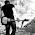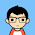Monday, July 26, 2010

Unification Algrithm.

UNIFICATION ALGORITHM

In propsoitional logic it is easy to determine that two literals can not both be true at the same time. Simply look for L and ~L . In predicate logic, this matching process is more complicated, since bindings of variables must be considered.

For example man (john) and man(john) is a contradiction while man (john) and man(Himalayas) is not. Thus in order to determine contradictions we need a matching procedure that compares two literals and discovers whether there exist a set of substitutions that makes them identical . There is a recursive procedure that does this matching . It is called Unification algorithm.

In Unification algorithm each literal is represented as a list, where first element is the name of a predicate and the remaining elements are arguments. The argument may be a single element (atom) or may be another list. For example we can have literals as

( tryassassinate Marcus Caesar)

( tryassassinate Marcus (ruler of Rome))

To unify two literals , first check if their first elements re same. If so proceed. Otherwise they can not be unified. For example the literals

( try assassinate Marcus Caesar)

( hate Marcus Caesar)

Can not be Unfied. The unification algorithm recursively matches pairs of elements, one pair at a time. The matching rules are :

i) Different constants , functions or predicates can not match, whereas identical ones can.

ii) A variable can match another variable , any constant or a function or predicate expression, subject to the condition that the function or [predicate expression must not contain any instance of the variable being matched (otherwise it will lead to infinite recursion).

iii) The substitution must be consistent. Substituting y for x now and then z for x later is inconsistent. (a substitution y for x written as y/x)

The Unification algorithm is listed below as a procedure UNIFY (L1, L2). It returns a list representing the composition of the substitutions that were performed during the match. An empty list NIL indicates that a match was found without any substitutions. If the list contains a single value F, it indicates that the unification procedure failed.

UNIFY (L1, L2)

1. if L1 or L2 is an atom part of same thing do

(a) if L1 or L2 are identical then return NIL

(b) else if L1 is a variable then do

(i) if L1 occurs in L2 then return F else return (L2/L1)

© else if L2 is a variable then do

(i) if L2 occurs in L1 then return F else return (L1/L2)

else return F.

2. If length (L!) is not equal to length (L2) then return F.

3. Set SUBST to NIL

( at the end of this procedure , SUBST will contain all the substitutions used to unify L1 and L2).

4. For I = 1 to number of elements in L1 do

i) call UNIFY with the i th element of L1 and I’th element of L2, putting the result in S

ii) if S = F then return F

iii) if S is not equal to NIL then do

(A) apply S to the remainder of both L1 and L2

(B) SUBST := APPEND (S, SUBST) return SUBST.

1.2.3.